## Archive for the 'chaos' Category

27
Oct
07

### Magnetic Pendulum Strange Attractor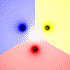This chaotic strange attractor represent the final resting positions for a magnetic pendulum suspended over some magnets (shown as black dots). It kind of looks like mixed paint. The 2D animation shows what happens as you decrease the damping factor. The 3D animation was shaded by path length.

Mathematica 4.2 version: 1/24/05; C++ version: 10/27/07; 3D rendered in POV-Ray 3.1

```(* runtime: 25 seconds, increase n for higher resolution *) n = 40; h = 0.25; g = 0.2; mu = 0.07; zlist = {Sqrt + I, -Sqrt + I, -2I}; image = Table[z2 = z /. NDSolve[{z''[t] == Plus @@ ((zlist - z[t])/(h^2 + Abs[zlist - z[t]]^2)^1.5) - g z[t] - mu z'[t], z == x + I y, z' == 0}, z, {t, 0, 25}, MaxSteps -> 200000][]; r = Abs[z2 - zlist]; i = Position[r, Min[r]][[1, 1]]; Hue[i/3], {y, -5.0, 5.0, 10.0/n}, {x, -5.0,5.0, 10.0/n}]; Show[Graphics[RasterArray[image]], AspectRatio -> 1];```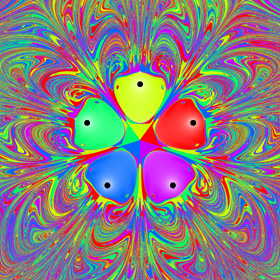The picture on the left shows another version with five magnets. See also my physics pendulums.

Here is some Mathematica code to numerically solve this using the Beeman integration scheme with the predictor-corrector modification:
```(* runtime: 45 seconds, increase n for higher resolution *) n = 40; tmax = 25; dt = 0.1; h = 0.25; g = 0.2; mu = 0.07; zlist = {Sqrt + I, -Sqrt + I, -2I}; image = Table[z = x + I y; v = a = a1 = 0; Do[z += v dt + (4a - a1)dt^2/6; vpredict = v + (3a - a1)dt/2; a2 = Plus @@ ((zlist - z)/(h^2 + Abs[zlist - z]^2)^1.5) - g z - mu vpredict; v += (2a2 + 5a - a1)dt/6; a1 =a; a = a2, {t, 0, tmax, dt}]; r = Abs[z - zlist]; Hue[Position[r, Min[r]][[1, 1]]/3], {y, -5.0, 5.0, 10.0/n}, {x, -5.0, 5.0, 10.0/n}]; Show[Graphics[RasterArray[image]], AspectRatio -> 1];```

16
Nov
06

### Driven Cavity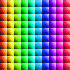Here is the flow inside a square box where the flow across the top of the box is moving to the right at Reynolds number 1000. This program uses the finite volume method to solve the Navier-Stokes equations assuming steady, incompressible, viscous, laminar flow. This was calculated on a 300×300 non-uniform grid and took 3 hours to run on my laptop. The animation shows the motion along the streamlines/pathlines.

Driven Cavity – calculated in Fortran 90, plotted in Mathematica 4.2, 11/2/05

The following Mathematica code is not completely accurate at the boundaries, but it gives the basic idea:

```(* runtime: 20 minutes *) n = 50; dx = 1.0/(n - 1); RE = 1000; relax = 0.4; residu = residv = residp = 1; u = Table[If[j == 1 || i == 2 || i == n, 0, 1], {i, 1, n}, {j, 1, n}]; v = p = pstar = ap = an = as = aw = ae = sc = sp = du = dv = Table[0, {n}, {n}]; lisolv[i0_, j0_, phi0_] := Module[{phi = phi0, p = q =Table[0, {n}]}, Do[q[[j0 - 1]] = phi[[i, j0 - 1]]; Do[p[[j]] = an[[i, j]]/(ap[[i, j]] -as[[i, j]]p[[j - 1]]); q[[j]] = (as[[i, j]]q[[j - 1]] + ae[[i, j]] phi[[i + 1, j]] + aw[[i, j]]phi[[i - 1, j]] + sc[[i, j]])/(ap[[i, j]] - as[[i, j]]p[[j - 1]]), {j, j0, n - 1}]; Do[phi[[i,j]] = p[[j]]phi[[i, j + 1]] + q[[j]], {j, n - 1, j0, -1}], {i, i0, n - 1}]; phi]; calc[i0_, j0_] := Module[{}, {cn, cs, ce, cw} = 0.5 {v[[i,j + 1]] + v[[i0, j0 + 1]], v[[i, j]] + v[[i0, j0]], u[[i + 1, j]] + u[[i0 + 1, j0]], u[[i, j]] + u[[i0, j0]]}dx; cp = Max[0, cn - cs + ce - cw]; {an[[i, j]], as[[i, j]], ae[[i, j]], aw[[i, j]]} = Map[Max[0, 1 - 0.5 Abs[#]] &, RE{cn, cs, ce, cw}]/RE + Map[Max[0, #] &, {-cn, cs, -ce, cw}]; sp[[i, j]] = -cp; ap[[i, j]] = an[[i, j]] + as[[i, j]] + ae[[i, j]] + aw[[i, j]] - sp[[i, j]]]; While[Max[residu, residv, residp] > 0.001, residu = residv = residp = 0; Do[calc[i - 1, j]; sc[[i, j]] = cp u[[i, j]] + dx(p[[i - 1, j]] - p[[i, j]]); du[[i, j]] = relax dx/ap[[i, j]]; residu += Abs[an[[i, j]]u[[i, j + 1]] + as[[i, j]]u[[i, j - 1]] + ae[[i, j]]u[[i + 1, j]] + aw[[i, j]]u[[i - 1, j]] - ap[[i, j]]u[[i, j]] + sc[[i, j]]], {i, 3, n - 1}, {j, 2, n - 1}]; ap /= relax; sc += (1 - relax) ap u; u = lisolv[3, 2, u]; Do[calc[i, j - 1]; sc[[i, j]] = cp v[[i, j]] + dx(p[[i, j - 1]] - p[[i, j]]); dv[[i, j]] = relax dx/ap[[i, j]];residv += Abs[an[[i, j]]v[[i, j + 1]] + as[[i, j]]v[[i, j - 1]] + ae[[i, j]]v[[i + 1, j]] + aw[[i, j]]v[[i - 1, j]] - ap[[i, j]]v[[i, j]] + sc[[i, j]]], {i, 2, n - 1}, {j, 3, n - 1}]; ap /= relax; sc += (1 - relax)ap v; v = lisolv[2, 3, v];Do[an[[i, j]] = dx dv[[i, j + 1]]; as[[i, j]] = dx dv[[i, j]]; ae[[i, j]] = dx du[[i + 1, j]]; aw[[i, j]] = dx du[[i, j]]; sp[[i, j]] = 0; sc[[i, j]] = -((v[[i, j + 1]] - v[[i, j]])dx + (u[[i + 1, j]] - u[[i, j]]) dx); residp += Abs[sc[[i, j]]]; ap[[i, j]] = an[[i, j]] + as[[i, j]] + ae[[i, j]] + aw[[i, j]] - sp[[i, j]]; pstar[[i, j]] = 0, {i, 2, n - 1}, {j, 2, n - 1}]; Do[pstar = lisolv[2, 2, pstar], {2}]; Do[If[i != 2, u[[i, j]] += du[[i, j]](pstar[[i - 1, j]] - pstar[[i, j]])]; If[j != 2, v[[i, j]] += dv[[i, j]](pstar[[i, j - 1]] - pstar[[i, j]])]; p[[i, j]] += 0.3(pstar[[i, j]] - pstar[[n - 1, n - 1]]), {i, 2, n - 1}, {j, 2, n - 1}]];```Here is some Mathematica code to plot streamlines. The blue lines are rotating clockwise and the red lines are rotating counter-clockwise. The plot on the left agrees fairly well with Ercan Erturk’s results.
```psi = Table[0, {n - 1}, {n - 1}]; Do[psi[[i, j]] = psi[[i, j - 1]] + dx(u[[i + 1, j - 1]] + u[[i + 1, j]])/2, {j, 2, n - 1}, {i, 1, n - 1}]; psi = ListInterpolation[psi, {{0, 1}, {0, 1}}]; ContourPlot[psi[x, y], {x, 0, 1}, {y, 0, 1}, PlotPoints -> 50, PlotRange -> All, ContourShading -> False,Contours -> {-0.08, -0.077, -0.07, -0.06, -0.045, -0.025, -0.01, -0.0025, 0, -8*^-6, 2*^-6, 3*^-5, 8*^-5, 1*^-4, 3*^-4, 6*^-4, 9*^-4}, ContourStyle -> Table[{Hue[2(1 - x)/3]}, {x, 0, 1, 1/16}]]```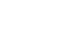Here is some Mathematica code to plot vorticity contours. The plot on the left agrees fairly well with Ghia’s results.
```omega = ListInterpolation[Table[(v[[i, j]] - v[[i - 1, j]])/dx - (u[[i, j]] - u[[i, j - 1]])/dx, {i, 2, n - 1}, {j, 2, n - 1}], {{0, 1}, {0, 1}}]; ContourPlot[omega[x, y], {x, 0, 1}, {y, 0, 1},PlotPoints -> 50, PlotRange -> All, ContourShading -> False, Contours -> Range[-14, 7],ContourStyle -> Table[{Hue[2(1 - x)/3]}, {x, 0, 1, 1/21}]]```

### Driven Cavity – Mathematica 4.2, 11/16/06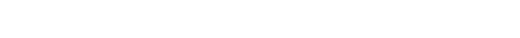Here is the same driven cavity using the finite difference method. This code uses the Alternating Direction Implicit (ADI) method to solve the vorticity transport equation assuming unsteady, incompressible, viscous flow.
```(* runtime: 1 minute *) << LinearAlgebra`Tridiagonal`; n = 20; RE = 1000; dx = 1.0/(n - 1); dt = 0.25; cxy = dt/(4dx); sxy = dt/(2RE dx^2); lambda = 1.5; free = Range[2, n - 1]; omega = v = psi = Table[0, {n}, {n}]; u = Table[If[j == n, 1, 0], {n}, {j, 1, n}]; Do[omega[[All, 1]] = 2psi[[All, 2]]/dx^2; omega[[All, n]] = 2 (psi[[All, n - 1]] + dx)/dx^2; omega[[1, All]] = 2 psi[[2, All]]/dx^2; omega[[n, All]] = 2 psi[[n - 1, All]]/dx^2; omega[[free, free]] = Transpose[Partition[TridiagonalSolve[Drop[Flatten[Table[If[i == n - 1, 0, -(cxy u[[i, j]] + sxy)], {j, 2, n - 1}, {i, 2, n - 1}]], -1], Flatten[Table[1 + 2sxy, {j, 2, n - 1}, {i, 2, n - 1}]], Drop[Flatten[Table[If[i == n - 1,0, cxy u[[i + 1, j]] - sxy], {j, 2,n - 1}, {i, 2, n - 1}]], -1], Flatten[Table[(cxy v[[i, j - 1]] + sxy)omega[[i, j - 1]] + (1 - 2sxy)omega[[i, j]] + (-cxy v[[i, j + 1]] + sxy)omega[[i, j + 1]] + If[i == 2, (cxy u[[i - 1, j]] + sxy)omega[[i - 1, j]],0] - If[i == n - 1, (cxy u[[i + 1, j]] - sxy) omega[[i + 1, j]], 0], {j, 2, n - 1}, {i, 2, n - 1}]]], n - 2]]; omega[[free, free]] = Partition[TridiagonalSolve[Drop[Flatten[Table[If[j == n - 1, 0, -(cxy v[[i, j]] + sxy)], {i, 2, n - 1}, {j, 2, n - 1}]], -1], Flatten[Table[1 + 2sxy, {i, 2, n - 1}, {j, 2, n - 1}]], Drop[Flatten[Table[If[j == n - 1, 0, cxy v[[i, j + 1]] - sxy], {i, 2, n - 1}, {j, 2, n - 1}]], -1], Flatten[Table[(cxy u[[i - 1, j]] + sxy)omega[[i - 1, j]] + (1 - 2sxy)omega[[i, j]] + (-cxy u[[i + 1, j]] +sxy)omega[[i + 1, j]] + If[j == 2, (cxy v[[i, j - 1]] + sxy)omega[[i, j - 1]], 0] - If[j == n - 1, (cxy v[[i, j + 1]] - sxy) omega[[i, j + 1]], 0], {i, 2, n - 1}, {j, 2, n - 1}]]], n - 2]; resid = 1; While[resid > 0.001, resid = 0; Do[resid += Abs[psi[[i, j]] - (psi[[i,j]] = lambda(psi[[i, j - 1]] + psi[[i - 1, j]] + psi[[i + 1, j]] + psi[[i, j + 1]] - dx^2omega[[i, j]])/4 + (1 - lambda)psi[[i, j]])], {i, 2, n - 1}, {j, 2, n - 1}]]; Do[u[[i, j]] = (psi[[i, j + 1]] - psi[[i, j - 1]])/(2dx); v[[i, j]] = -(psi[[i + 1, j]] - psi[[i - 1, j]])/(2dx), {i, 2, n - 1}, {j, 2, n - 1}]; ListContourPlot[Transpose[psi], PlotRange -> All, ContourShading -> False], {100}];```

Link: Driven Cavity – simple Fortran program by Zheming Zheng that uses this method

10
Apr
06

### Lorenz AttractorThe Lorenz Attractor is a famous chaotic strange attractor. The equations were originally developed to model atmospheric convection.
```(* runtime: 1 second *) sigma = 3; rho = 26.5; beta = 1; soln = {x[t], y[t], z[t]} /. NDSolve[{x'[t] == sigma (y[t] - x[t]), y'[t] == rho x[t] - x[t]z[t] - y[t], z'[t] == x[t] y[t] - beta z[t], x == 0, y == 1, z == 1}, {x[t], y[t], z[t]}, {t, 0, 100}, MaxSteps -> 10000][]; ParametricPlot3D[soln, {t, 0, 100}, PlotPoints -> 10000, Compiled -> False];```

Here is some Mathematica code to numerically solve this using the 4th order Runge-Kutta method:
(* runtime: 0.2 second *)
```sigma = 3; rho = 26.5; beta = 1; dt = 0.01; p = {0, 1, 1}; v[{x_, y_, z_}] = {sigma(y - x), rho x - x z - y, x y - beta z}; Show[Graphics3D[Line[Table[k1 = dt v[p]; k2 = dt v[p + k1/2]; k3 = dt v[p +k2/2]; k4 = dt v[p + k3]; p += (k1 + 2 k2 + 2 k3 + k4)/6, {1000}]]]];```

## Welcome !

You will find here some of my favorite hobbies and interests, especially science and art.

I hope you enjoy it!

Subscribe to theRSS feed to stay informed when I publish something new here.

I would love to hear from you! Please feel free to send me an email : bugman123-at-gmail-dot-com

## TopicsBerna Blalack on Magnetic Pendulum Strange…Daan on Magnetic Pendulum Strange…Sebastian Schepis on Diffusion Limited Aggregation…mohammad_andito on CFM56-5 Turbofan Jet Engi…SasQ on Magnetic Field of a Solen… OUPblog » Blog… on Diamond Light Dispersion Complex Roots on Polynomial Roots Joukowski airfoils |… on Joukowski AirfoilKarim Alame on Flapping WingREJISH J on Joukowski Airfoil SOLINOID | Materials… on Magnetic Field of a Solen…Emanuele on 4D “Squarry” Julia…Emanuele on Hydrogen Electron Orbital Prob…Tim on Mandelbrot Set Pickover S…khankasi1 on 4D Quaternion Mandelbrot …